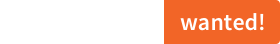# sciss / equal

Simple macro-based type safe equals operator ===. Mirror of https://git.iem.at/sciss/Equal

GitHub# Equal

## statement

Equal is a small library for Scala to provide zero-overhead type-safe equals and not-equals operators `===` and `!==`.

It is (C)opyright 2016–2017 by Hanns Holger Rutz. All rights reserved. Equal is released under the GNU Lesser General Public License v2.1+ and comes with absolutely no warranties. To contact the author, send an email to `contact at sciss.de`.

Warning: This is not yet thoroughly tested.

## requirements / installation

This project compiles against Scala 2.12, 2.11, 2.10 using sbt 0.13. Note: Under Scala 2.10, the macro is just a dummy implementation that does not actually perform a type check. So your project should at least be cross-compiled including Scala 2.11 or 2.12!

To use the library in your project:

``````"de.sciss" %% "equal" % v
``````

The current version `v` is `"0.1.2"`

## documentation

You import the operators using

``````import de.sciss.equal.Implicits._
``````

Then the following compiles:

``````Vector(1, 2, 3) === List(1, 2, 3)
"hello" !== "world"
4 !== 5
Option("foo") !== None
List(Some(1), None) === Seq(Some(1), None)

def contains[A](in: Option[A], elem: A): Boolean = in === Some(elem)
``````

While the following does not:

``````Vector(1, 2, 3) === Set(1, 2, 3)    // to-do: mysterious error message
List(1, 2) === ((1, 2))
4 !== 5f
"hello" === Some("hello")

def contains[A, B](in: Option[A], elem: B): Boolean = in === Some(elem)
``````